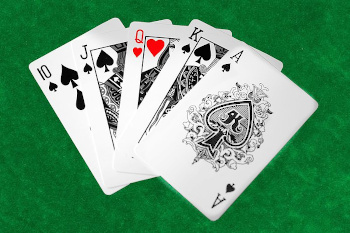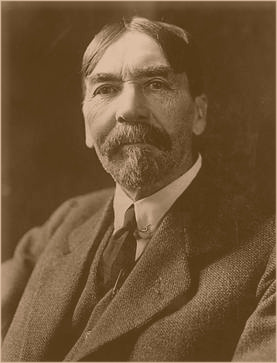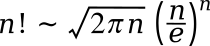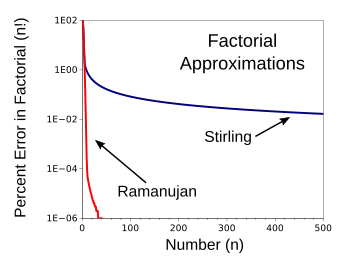### Factorials

December 14, 2020

Mathematicians are reserved people who generally stay in quiet rooms for extended periods as they scratch out their latest proof with pencil on paper. Experimental mathematicians are somewhat more agitated, since they are stimulated by the glow of their display screens and the hum of their computer fan. I've heard that the proof of Fermat's last theorem was celebrated by adding an extra teaspoonful of honey to their teacups at afternoon tea.

That's why I was surprised as a student to learn that the symbol for the factorial operation was an exclamation point. The factorial N! of a number, N, is the product of all positive integers less than or equal to N; that is, (N)(N-1)(N-2)...(1). As an example, 5! is (5)(4)(3)(2)(1) = 120, and 0! is arbitrarily defined as equal to 1.

Mathematics developed because of its utility in solving problems in ordinary life, such as calculating land areas for taxes. The Greek philosopher, Thales of Miletus (c. 624-c. 545 BC), used geometry to determine the distance to ships from a shoreline, which was the same method of proportional triangles that I learned as a Boy Scout for finding the width of a river. Renaissance mathematicians took an interest in the calculation of gambling odds, such as the probability of rolling certain number sums with dice, and that's when factorials became useful.In the simple days of my childhood (BC, as in "before computers"), there weren't many things to occupy a child's time; so, my brothers and I would often play card games.

Shown here is a straight poker hand, Ace high. If the Queen of Hearts were instead a Queen of Spades, the hand would be a Royal Flush, the best hand in Poker. The probability of being dealt such a hand is just 0.000154% (see below). (Wikimedia Commons image by sunkiddance.)

There are two operations involving factorials that are used in determining probabilities; namely, combinations and permutations. When the order of selecting objects doesn't matter, we use a combination, and when the order is important, we use a permutation. A combination of k objects taken from a collection of n objects, C(n,k), is given as (n!)/(k!(n-k)!), and the permutation of n things selected from k choices, P(n,k), is given as (n!)/((n-k)!). One interesting fact is that what's called a combination lock is actually a permutation lock.

As an example of a combination, let's say you've just had a bad week, and you were interested in how each week in your year of 52 weeks relates to another. You would calculate the combination of 52 things taken two at a time, C(52,2) = (52!)/(2!(52-2)!) = 1,326. Likewise for poker hands, the probability of drawing a particular five card hand from the deck of 52 unique cards (thirteen different values in four suits) would be C(52,5) = (52!)/(5!(52-5)!) = one chance out of 2,598,960, or 0.000038477%.

There are four different ways to get a Royal Flush, since there are four suits, so this probability can be multiplied by four to give a 0.000154% chance of being dealt a Royal Flush. As an example of permutations, perhaps you want to create a composite month of four weeks from the 52 weeks of the year. There are P(52,4) = 52!/(52-4)! = 6,497,400 different ways you can do this.

The factorial of numbers blows up quite quickly, as the following table shows.

N N!
1 1
10 3.628800 x 106
100 9.332621544 x 10157
1000 4.023872601 x 102567
10000 2.846259681 x 1035659
100000 2.824229408 x 10456573
1000000 8.263931688 x 105565708

Excel and Gnumeric can only calculate factorials up to 170! = 7.257416e+306. This is also the limit for MATLAB, a computation and graphing application used by many researchers. I don't use MATLAB, or the similar Mathematica application, since I'm an advocate for free and open source software (FOSS). I'm as impressed by the high cost of these applications as I am by their functionality.

It's unfortunate that software companies have embraced a business model in which they rent, rather than sell, their software. The economist, Thorstein Veblen (a.k.a., Torsten Veblen), wrote about "charging what the market will bear" in his 1899 book, The Theory of the Leisure Class.Thorstein (Torsten) Veblen (1857-1929).

Veblen's "The Theory of the Leisure Class" introduced the term, "conspicuous consumption."

Conspicuous consumption is the purchase of lavishly expensive, or ephemeral and impractical, goods and services simply as a way of displaying income or wealth.

(Wikimedia Commons image, modified for artistic effect)

Although I use the PHP language for simple tasks, my usual computation language for mathematics is the C programming language. C code, compiled using the GCC compiler and a long long int type for N will only give factorials up to 20! = 2432902008176640000 (2.4329 x 1018). However, there is a library that allows much larger factorials than this.

The GNU Multiple Precision Arithmetic Library (GMP) is easily installed on a Linux computer. This library extends our access to large numbers considerably, although there are limits imposed by memory space on a desktop computer. I haven't used GMP in more than a decade, but I was able to hack together a program to calculate factorials, and also the approximation equation for factorials developed by mathematician, James Stirling (1692-1770) (Source code here). Another approximation, as described below, gives much better values, and I coded this in the program, also.

Of course, it's tedious to multiply many numbers together, especially when you don't need a precise result. That's why Stirling's factorial approximation equation is useful. As sometimes happens in science and mathematics, this equation was named after Stirling, although it was first stated by Abraham de Moivre (1667-1754). De Moivre was interested in probabilities, and he was the author of the first textbook on probability, The Doctrine of Chances (1718). Stirling's equation, asymptotic to the actual value of n! (it's better as n gets larger), is as follows:Centuries later, preeminent number theorist, Srinivasa Ramanujan (1887-1920), discovered a more precise approximation. This was written only in a notebook, but it was eventually published in 1988.So, how much better is Ramanujan's approximation than Stirling's? One glance at the following graph, derived from data generated by my evaluation program, tells all. The percentage error of Ramanujan's approximation at n=500 is just 8.1958 x 10-7Stirling's Factorial Approximations, compared to the approximation created by Ramanujan, as calculated by my computer program.

Ramanujan's simple equation wins by a wide margin.

(Graphed using Gnumeric### References:

Linked Keywords: Mathematician; reserved; room; mathematical proof; pencil; paper; experimental mathematics; experimental mathematician; agitated; stimulated; computer monitor; display screen; hum; computer fan; Fermat's last theorem; party; celebrate; teaspoon; teaspoonful; honey; teacups; afternoon tea; student; mathematical symbol; factorial; operation (mathematics); exclamation mark; exclamation point; natural number; product (mathematics); positive integer; calculation; calculating; land; area; tax; Ancient Greek philosophy; Greek philosopher; Thales of Miletus (c. 624-c. 545 BC); geometry; distance; ship; shoreline; proportionality (mathematics); proportional; triangle; Boy Scouts of America; width; river; Renaissance; fixed-odds betting; gambling odds; poker probability; dice roll; addition; sum; dice; childhood; computer; brother; card game; straight poker hand, Ace high; Queen (playing card); Queen of Hearts; Queen of Spades; Royal Flush; card game dealing; Wikimedia Commons; sunkiddance; combination; permutation; hierarchy; order; combination lock; week; year; poker probability; poker hand; playing card suit; straight flush; Royal Flush; composite; month; Excel; Gnumeric; MATLAB; computation; chart; graph; application software; research; researcher; Mathematica; free and open source software (FOSS); cost; software company; business model; rent; sell; economist; Thorstein Veblen; The Theory of the Leisure Class; Thorstein (Torsten) Veblen (1857-1929); conspicuous consumption; lavishly expensive; ephemeral; goods; service (economics); income; wealth; PHP language; C programming language; GNU Compiler; C data type; long long int type; library (computing); GNU Multiple Precision Arithmetic Library (GMP); Linux; computer memory; memory space; desktop computer; decade; Stirling's approximation equation for factorials; James Stirling (1692-1770); stirling ramanujan.c; accuracy and precision; precise; science; Abraham de Moivre (1667-1754); author; textbook; The Doctrine of Chances; asymptote; asymptotic; century; greatness; preeminent; number theory; number theorist; Srinivasa Ramanujan (1887-1920); notebook; academic publishing; published<; Cartesian coordinate system; data.